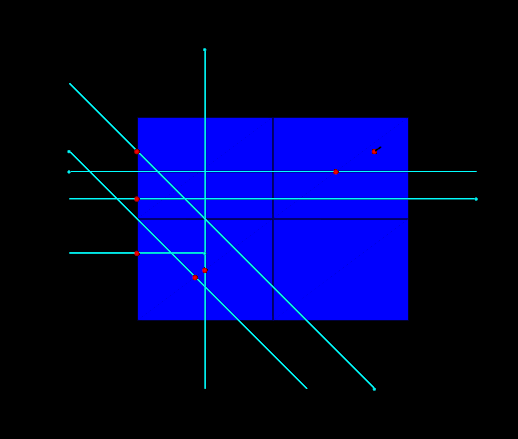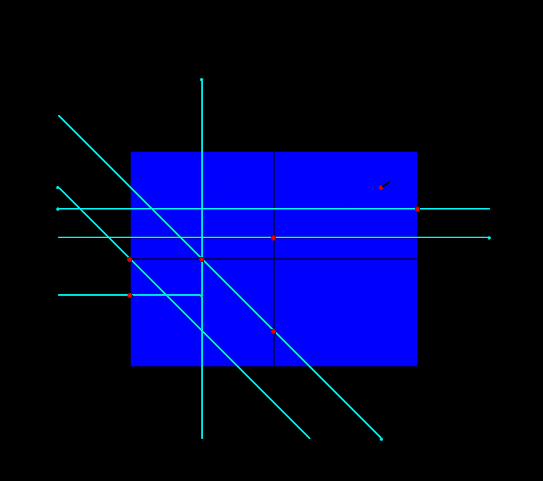# vtkCellLocator::IntersectWithLine is unreliable in 2D

I want to find the intersection point on a surface of a line, where the line and the surface are both in the Z=0 plane. I would hope/expect that vtkCellLocator::IntersectWithLine always finds a point on the edge of the surface, closest to the starting point of the line, however this does not always seem to be the case. Also the behavior of the routine changed from VTK 9.1 to VTK 9.2. Is this an error or unintended use of the vtkCellLocator. If the latter is the case, is there an alternative to use for 2D situations?

I include a small script (intersect_with_line_2D.py (3.4 KB)) to demonstrate the behavior for some in-plane lines and one line (in the upper right corner) that runs in the Z-direction.

The lines are directed towards the dot, and the corresponding intersection point found by the cell locator is shown in red.

VTK 9.1VTK 9.2Output of VTK 9.2

``````sheet bounds=(0.0, 4.0, 0.0, 3.0, 0.0, 0.0)
line=((3.5, 2.5, 1), (3.5, 2.5, -1)) success=1 t=0.0 x=[3.5, 2.5, 0.0] pcoords=[0.6666666666666667, 0.75, 0.0] subId=0 cellId=0
line=((2.5, -1.0, 0), (-1, 2.5, 0)) success=1 t=0.0 x=[0.0, 1.5, 0.0] pcoords=[-0.0, -0.0, 0.0] subId=0 cellId=0
line=((-1, 3.5, 0), (3.5, -1, 0)) success=1 t=0.0 x=[2.0, 0.5, 0.0] pcoords=[0.3333333333333333, -0.0, 0.0] subId=0 cellId=0
line=((1, -1, 0), (1, 4, 0)) success=1 t=0.0 x=[1.0, 1.5, 0.0] pcoords=[0.0, 0.5, 0.0] subId=0 cellId=0
line=((-1, 1, 0), (1, 1, 0)) success=1 t=0.0 x=[0.0, 1.0, 0.0] pcoords=[0.6666666666666666, -0.0, 0.0] subId=0 cellId=0
line=((-1, 1.8, 0), (5, 1.8, 0)) success=1 t=0.0 x=[2.0, 1.8, 0.0] pcoords=[0.20000000000000004, -0.0, 0.0] subId=0 cellId=0
line=((5, 2.2, 0), (-1, 2.2, 0)) success=1 t=0.0 x=[4.0, 2.2, 0.0] pcoords=[0.4666666666666668, 1.0, 0.0] subId=0 cellId=0
``````

Hello,

Mathematically, the only case when the intersection between a line and a polygon (a plane is infinite) in 2D is a point occurs when the line touches one of the polygon vertexes. From what is depicted, the intersections were supposed to be lines too. Hence, any point lying along the line and within the polygon is technically a valid result. I wouldn’t call that unreliable, but undefined behavior.

take care,

Paulo

use vtkcutter

I’m interested in the nearest intersection point of a ray on my surface. The example that I included contains a surface in 2D, some rays (the end of the ray is marked with a dot), and the intersection points that the rays produce.

In a 3D situation, the intersect-with-line function yields the nearest point where the line intersects a surface.

In the 2D situation I would expect that the function yields the nearest point on the edge of the surface, not a point on an inner edge as often, and randomly, happens. I think this is caused by numerical noise when evaluating the line intersection with each sub-cell.

Well, if it works in 3D as expected by you, then try to do it in 3D behind the scenes (use Z=constant for all points) and render the results in 2D by dropping the Z coordinate.

But again: the intersection between a line and a filled polygon is another line (not counting special or trivial cases). So, even in 3D, the current behavior may change in the future.

Perhaps you’re better off by using other functions to accomplish that: Get intersection of PolyData line and a plane